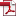Performance Analysis of Cellular Networks with D2D communication Based on Queuing Theory Model
• KSII Transactions on Internet and Information Systems
Monthly Online Journal (eISSN: 1976-7277)

# Performance Analysis of Cellular Networks with D2D communication Based on Queuing Theory Model

Vol. 12, No. 6, June 29, 201810.3837/tiis.2018.06.002 , Download Paper (Free):#### Abstract

In this paper, we develop a spatiotemporal model to analysis of cellular user in underlay D2D communication by using stochastic geometry and queuing theory. Firstly, by exploring stochastic geometry to model the user locations, we derive the probability that the SINR of cellular user in a predefined interval, which constrains the corresponding transmission rate of cellular user. Secondly, in contrast to the previous studies with full traffic models, we employ queueing theory to evaluate the performance parameters of dynamic traffic model and formulate the cellular user transmission mechanism as a M/G/1 queuing model. In the derivation, Embedded Markov chain is introduced to depict the stationary distribution of cellular user queue status. Thirdly, the expressions of performance metrics in terms of mean queue length, mean throughput, mean delay and mean dropping probability are obtained, respectively. Simulation results show the validity and rationality of the theoretical analysis under different channel conditions.